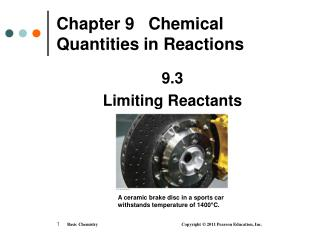DownloadDownload PresentationChapter 9 Chemical Quantities in Reactions

# Chapter 9 Chemical Quantities in Reactions

Télécharger la présentation## Chapter 9 Chemical Quantities in Reactions

- - - - - - - - - - - - - - - - - - - - - - - - - - - E N D - - - - - - - - - - - - - - - - - - - - - - - - - - -
##### Presentation Transcript

1. A ceramic brake disc in a sports car withstands temperature of 1400°C. Chapter 9 Chemical Quantities in Reactions 9.3 Limiting Reactants

2. Limiting Reactant A limiting reactant in a chemical reaction is the substance that • is used up first • stops the reaction • limits the amount of product that can form

3. Reacting Amounts In a table setting, there are 1 fork, 1 knife, and 1 spoon. How many table settings are possible from 6 forks, 4 spoons, and 7 knives? What is the limiting item?

4. Reacting Amounts Four table settings are possible. Initially Used Extra forks 6 4 2 spoons 4 4 0 knives 7 4 3 The limiting item is the spoon.

5. Example of Everyday Limiting Reactant How many peanut butter sandwiches can be made from 8 slices of bread and 1 jar of peanut butter? With 8 slices of bread, only 4 sandwiches can be made. The bread is the limiting reactant.

6. Example of Everyday Limiting Reactant How many peanut butter sandwiches can be made from 8 slices of bread and 1 tablespoon of peanut butter? With 1 tablespoon of peanut butter, only 1 sandwich can be made. The peanut butter is the limiting item.

7. Limiting Reactants When 4.00 mol of H2 is mixed with 2.00 mol of Cl2, how many moles of HCl can form? H2(g) + Cl2(g)  2HCl (g) 4.00 mol 2.00 mol ??? Mol

8. Limiting Reactants Using Moles Calculate the moles of product from each reactant, H2 and Cl2. moles of HCl from moles of H2 4.00 mol H2 x 2 mol HCl = 8.00 mol of HCl 1 mol H2 (not possible) moles of HCl from moles of Cl2 2.00 mol Cl2 x 2 mol HCl = 4.00 mol of HCl 1 mol Cl2 (smaller number) The limiting reactant is Cl2 because it produces the smaller number of moles of HCl.

9. Checking Calculations

10. Calculating Mass of Product from a Limiting Reactant

11. Limiting Reactants Using Mass Calculate the mass of water produced when 8.00 g of H2 and 24.0 g of O2 react? 2H2(g) + O2(g) 2H2O(l)

12. Limiting Reactants Using Mass STEP 1 Use molar mass to convert the grams of each reactant to moles. Given 8.00 g of H2 and 24.0 g of O2 Need grams of H2O 8.00 g H2 x 1 mol H2 = 3.97 mol of H2 2.016 g H2 24.0 g O2 x 1 mol O2 = 0.750 mol of O2 32.00 g O2

13. Limiting Reactants Using Mass (continued) STEP 2Write mole-mole factors using the coefficients in the equation. 1 mol of O2 = 2 mol of H2O 1 mol O2 and 2 mol H2O 2 mol H2O 1 mol O2 2 mol of H2 = 2 mol of H2O 2 mol H2 and 2 mol H2O 2 mol H2O2 mol H2

14. Limiting Reactants Using Mass (continued) STEP 3 Calculate moles of product from each reactant and determine the limiting reactant. moles of H2O from moles of H2 3.97 mol H2 x 2 mol H2O = 3.97 mol of H2O 2 mol H2 (not possible) moles of H2O from moles of O2 0.750 mol O2 x 2 mol H2O = 1.50 mol of H2O 1 mol O2 (smaller number) The limiting reactant is O2 because it produces the smaller moles (1.50 mol) of H2O.

15. Limiting Reactants Using Mass (continued) STEP 4 Determine the moles of product or calculate grams of product using molar mass. 1.50 mol H2O x 18.02 g H2O = 27.0 g of H2O 1 mol H2O